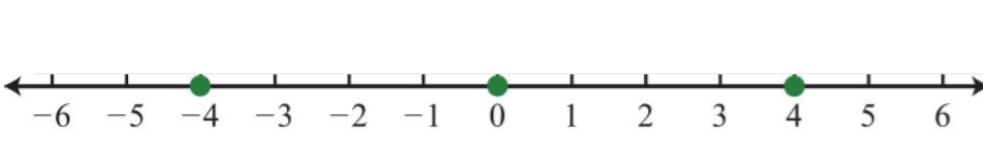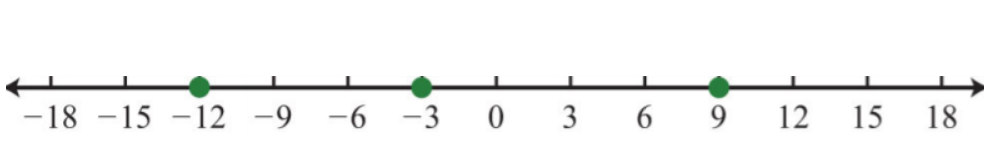$$\newcommand{\id}{\mathrm{id}}$$ $$\newcommand{\Span}{\mathrm{span}}$$ $$\newcommand{\kernel}{\mathrm{null}\,}$$ $$\newcommand{\range}{\mathrm{range}\,}$$ $$\newcommand{\RealPart}{\mathrm{Re}}$$ $$\newcommand{\ImaginaryPart}{\mathrm{Im}}$$ $$\newcommand{\Argument}{\mathrm{Arg}}$$ $$\newcommand{\norm}{\| #1 \|}$$ $$\newcommand{\inner}{\langle #1, #2 \rangle}$$ $$\newcommand{\Span}{\mathrm{span}}$$

# 1.E: Review Exercises and Sample Exam

$$\newcommand{\vecs}{\overset { \rightharpoonup} {\mathbf{#1}} }$$

$$\newcommand{\vecd}{\overset{-\!-\!\rightharpoonup}{\vphantom{a}\smash {#1}}}$$

## Review Exercises

Exercise $$\PageIndex{1}$$ Real Numbers and the Number Line

Choose an appropriate scale and graph the following sets of real numbers on a number line.

1. $$\{−4, 0, 4\}$$
2. $$\{−30, 10, 40\}$$
3. $$\{−12, −3, 9\}$$
4. $$\{−10, 8, 10\}$$

1.Figure 1.E.1

3.Figure 1.E.2

Exercise $$\PageIndex{2}$$ Real Numbers and the Number Line

Fill in the blank with $$<, =,\text{ or }>$$.

1. $$0\underline{\quad}-9$$
2. $$-75\underline{\quad}-5$$
3. $$-12\underline{\quad}-(-3)$$
4. $$-(-23)\underline{\quad}23$$
5. $$|-20|\underline{\quad}-|-30|$$
6. $$-|6|\underline{\quad}-|-(-8)|$$

1. $$>$$

3. $$<$$

5. $$>$$

Exercise $$\PageIndex{3}$$ Real Numbers and the Number Line

Determine the unknown.

1. $$|?|=2$$
2. $$|?|=1$$
3. $$|?|=-7$$
4. $$|?|=0$$

1. $$\pm 2$$

3. $$Ø$$, No solution

Exercise $$\PageIndex{4}$$ Real Numbers and the Number Line

Translate the following into a mathematical statement.

1. Negative eight is less than or equal to zero.
2. Seventy-eight is not equal to twelve.
3. Negative nine is greater than negative ten.
4. Zero is equal to zero.

1. $$-8\leq 0$$

3. $$-9>-10$$

Exercise $$\PageIndex{5}$$ Adding and Subtracting Integers

Simplify.

1. $$12+(−7)$$
2. $$20+(−32)$$
3. $$−23−(−7)$$
4. $$−8−(−8)$$
5. $$−3−(−13)+(−1)$$
6. $$9+(−15)−(−8)$$
7. $$(7−10)−3$$
8. $$(−19+6)−2$$

1. $$5$$

3. $$-16$$

5. $$9$$

7. $$-6$$

Exercise $$\PageIndex{6}$$ Adding and Subtracting Integers

Find the distance between the given numbers on a number line.

1. $$−8$$ and $$14$$
2. $$−35$$ and $$−6$$
3. What is $$2$$ less than $$17$$?
4. What is $$3$$ less than $$−20$$?
5. Subtract $$30$$ from the sum of $$8$$ and $$12$$.
6. Subtract $$7$$ from the difference of $$−5$$ and $$7$$.
7. An airplane flying at $$22,000$$ feet descended $$8,500$$ feet and then ascended $$5,000$$ feet. What is the new altitude of the airplane?
8. The width of a rectangle is $$5$$ inches less than its length. If the length measures $$22$$ inches, then determine the width.

1. $$22$$ units

3. $$15$$

5. $$-10$$

7. $$18,500$$ feet

Exercise $$\PageIndex{7}$$ Multiplying and Dividing Integers

Simplify.

1. $$10÷5⋅2$$
2. $$36÷6⋅2$$
3. $$−6(4)÷2(−3)$$
4. $$120÷(−5)(−3)(−2)$$
5. $$−8(−5)÷0$$
6. $$−24(0)÷8$$
7. Find the product of $$−6$$ and $$9$$.
8. Find the quotient of $$−54$$ and $$−3$$.
9. James was able to drive $$234$$ miles on $$9$$ gallons of gasoline. How many miles per gallon did he get?
10. If a bus travels at an average speed of $$54$$ miles per hour for $$3$$ hours, then how far does the bus travel?

1. $$4$$

3. $$36$$

5. Undefined

7. $$-54$$

9. $$26$$ miles per gallon

Exercise $$\PageIndex{8}$$ Fractions

Reduce each fraction to lowest terms.

1. $$\frac{180}{300}$$
2. $$\frac{252}{324}$$
3. Convert to a mixed number: $$\frac{23}{8}$$.
4. Convert to an improper fraction: $$3\frac{5}{9}$$.

1. $$\frac{3}{5}$$

3. $$2\frac{7}{8}$$

Exercise $$\PageIndex{9}$$ Fractions

Simplify.

1. $$\frac{3}{5}(−\frac{2}{7})$$
2. $$−\frac{5}{8}(−\frac{1}{3})$$
3. $$−\frac{3}{4}÷\frac{6}{7}$$
4. $$\frac{4}{15}÷\frac{28}{3}$$
5. $$4\frac{4}{5}÷6$$
6. $$5÷8\frac{1}{3}$$
7. $$\frac{5}{4}÷\frac{15}{2}⋅6$$
8. $$\frac{5}{24}÷\frac{3}{2}÷\frac{5}{12}$$
9. $$\frac{1}{12}−\frac{1}{4}$$
10. $$\frac{5}{6}−\frac{3}{14}$$
11. $$\frac{3}{4}+\frac{2}{3}−\frac{1}{12}$$
12. $$\frac{3}{10}+\frac{5}{12}−\frac{1}{6}$$
13. Subtract $$\frac{2}{3}$$ from the sum of $$−\frac{1}{2}$$ and $$\frac{2}{9}$$.
14. Subtract $$\frac{5}{6}$$ from the difference of $$\frac{1}{3}$$ and $$\frac{7}{2}$$.
15. If a bus travels at an average speed of $$54$$ miles per hour for $$2\frac{1}{3}$$ hours, then how far does the bus travel?
16. Determine the length of fencing needed to enclose a rectangular pen with dimensions $$12\frac{1}{2}$$ feet by $$8\frac{3}{4}$$ feet.

1. $$-\frac{6}{35}$$

3. $$-\frac{7}{8}$$

5. $$\frac{4}{5}$$

7. $$1$$

9. $$-\frac{1}{6}$$

11. $$\frac{4}{3}$$

13. $$-\frac{17}{18}$$

15. $$126$$ miles

Exercise $$\PageIndex{10}$$ Decimals and Percents

1. Write as a mixed number: $$5.32$$.
2. Write as a decimal: $$7\frac{3}{25}$$

1. $$5\frac{8}{25}$$

Exercise $$\PageIndex{11}$$ Decimals and Percents

Perform the operations.

1. $$6.032+2.19$$
2. $$12.106−9.21$$
3. $$4.23×5.13$$
4. $$9.246÷4.02$$

1. $$8.222$$

3. $$21.6999$$

Exercise $$\PageIndex{12}$$ Decimals and Percents

Convert to a decimal.

1. $$7.2$$%
2. $$5\frac{3}{8}$$%
3. $$147$$%
4. $$27\frac{1}{2}$$%

1. $$0.072$$

3. $$1.47$$

Exercise $$\PageIndex{13}$$ Decimals and Percents

Convert to a percent.

1. $$0.055$$
2. $$1.75$$
3. $$\frac{9}{10}$$
4. $$\frac{5}{6}$$
5. Mary purchased $$3$$ boxes of t-shirts for a total of $$$126$$. If each box contains $$24$$ t-shirts, then what is the cost of each t-shirt? 6. A retail outlet is offering $$12$$% off the original$$$39.99$$ price of tennis shoes. What is the price after the discount?
7. If an item costs $$$129.99$$, then what is the total after adding $$7\frac{1}{4}$$% sales tax? 8. It is estimated that $$8.3$$% of the total student population carpools to campus each day. If there are $$13,000$$ students, then estimate the number of students that carpool to campus. Answer 1. $$5.5$$% 3. $$90$$% 5.$$$1.75$$

7. \$$$139.41$$

Exercise $$\PageIndex{14}$$ Exponents and Square Roots

Simplify.

1. $$8^{2}$$
2. $$(−5)^{2}$$
3. $$−4^{2}$$
4. $$−(−3)^{2}$$
5. $$(\frac{2}{9})^{2}$$
6. $$(1\frac{2}{3})^{2}$$
7. $$3^{3}$$
8. $$(−4)^{3}$$
9. $$(\frac{2}{5})^{3}$$
10. $$(−\frac{1}{6})^{3}$$
11. $$−(−2)^{4}$$
12. $$−(−1)^{5}$$
13. $$\sqrt{49}$$
14. $$\sqrt{225}$$
15. $$2\sqrt{25}$$
16. $$−\sqrt{121}$$
17. $$3\sqrt{50}$$
18. $$−4\sqrt{12}$$
19. $$4\sqrt{9}$$
20. $$8\sqrt{25}$$
21. Calculate the area of a square with sides measuring $$3$$ centimeters. $$(A=s^{2})$$
22. Calculate the volume of a cube with sides measuring $$3$$ centimeters. $$(V=s^{3})$$
23. Determine the length of the diagonal of a square with sides measuring $$3$$ centimeters.
24. Determine the length of the diagonal of a rectangle with dimensions $$2$$ inches by $$4$$ inches.

1. $$64$$

3. $$−16$$

5. $$\frac{4}{81}$$

7. $$27$$

9. $$\frac{81}{25}$$

11. $$−16$$

13. $$7$$

15. $$10$$

17. $$15\sqrt{2}$$

19. $$\frac{2}{3}$$

21. $$9$$ square centimeters

23. $$3\sqrt{2}$$ centimeters

Exercise $$\PageIndex{15}$$ Order of Operations

Simplify.

1. $$−5(2)−7^{2}$$
2. $$1−4^{2}+2(−3)^{2}$$
3. $$2+3(6−2⋅4)^{3}$$
4. $$5−3(8−3⋅4)^{2}$$
5. $$−2^{3}+6(3^{2}−4)+(−3)^{2}$$
6. $$5^{2}−40÷5(−2)^{2}−(−4)$$
7. $$\frac{3}{4}[\frac{2}{9}(−3)^{2}−4]^{2}$$
8. $$(\frac{1}{2})^{2}−\frac{3}{4}÷\frac{9}{16}−\frac{1}{3}$$
9. $$\frac{2−3(6−3^{2})^{2}}{4⋅5−5^{2}}$$
10. $$\frac{(2⋅8−6^{2})^{2}−10}{273−(2(−5)^{3}−7) }$$
11. $$8−5|3⋅4−(−2)^{4}|$$
12. $$|14|-|−3−52|$$

1. $$-59$$

3. $$-22$$

5. $$31$$

7. $$3$$

9. $$5$$

11. $$-12$$

Exercise $$\PageIndex{16}$$ Order of Operations

Find the distance between the given numbers on a number line.

1. $$−14$$ and $$22$$
2. $$−42$$ and $$−2$$
3. $$\frac{7}{8}$$ and $$−\frac{1}{5}$$
4. $$−5\frac{1}{2}$$ and $$−1\frac{1}{4}$$

1. $$36$$ units

3. $$\frac{43}{40}$$ units

## Sample Exam

Exercise $$\PageIndex{17}$$

1. List three integers greater than $$−10$$.
2. Determine the unknown(s): $$| ? |=13$$.
3. Fill in the blank with $$<,=,\text{ or }$$ : $$-|-100|\underline{\:\:}9^{2}$$.
4. Convert to a fraction: $$33\frac{1}{3}$$%
5. Convert to a percent: $$2\frac{3}{4}$$.
6. Reduce: $$\frac{75}{225}$$.

1. $$\{−5, 0, 5\}$$ (answers may vary)

3. $$<$$

5. $$275$$%

Exercise $$\PageIndex{18}$$

Calculate the following.

1. a. $$(−7)^{2}$$; b. $$−(−7)^{2}$$; c. $$−7^{2}$$
2. a. $$(−3)^{3}$$; b. $$−(−3)^{3}$$; c. $$−3^{3}$$
3. a. $$|10|$$; b. $$−|−10|$$; c. $$−|10|$$

1. a. $$49$$; b. $$−49$$; c. $$−49$$

3. a. $$10$$; b. $$−10$$; c. $$−10$$

Exercise $$\PageIndex{19}$$

Simplify.

1. $$−(−(−1))$$
2. $$\frac{2}{3}+\frac{1}{5}−\frac{3}{10}$$
3. $$10−(−12)+(−8)−20$$
4. $$−8(4)(−3)÷2$$
5. $$\frac{1}{2}⋅(−\frac{4}{5})÷\frac{14}{15}$$
6. $$\frac{3}{5}⋅\frac{1}{2}−\frac{2}{3}$$
7. $$4⋅5−20÷5⋅2$$
8. $$10−7(3−8)−5^{2}$$
9. $$3+2|−2^{2}−(−1)|+(−2)^{2}$$
10. $$\frac{1}{3}[5^{2}−(7−|−2|)+15⋅2÷3]$$
11. $$\sqrt{116}$$
12. $$3\sqrt{72}$$

2. $$\frac{17}{30}$$

4. $$48$$

6. $$-\frac{11}{30}$$

8. $$20$$

10. $$10$$

12. $$18\sqrt{2}$$

Exercise $$\PageIndex{20}$$

1. Subtract $$2$$ from the sum of $$8$$ and $$−10$$.
2. Subtract $$10$$ from the product of $$8$$ and $$−10$$.
3. A student earns $$9, 8, 10, 7,$$ and $$8$$ points on the first $$5$$ chemistry quizzes. What is her quiz average?
4. An $$8\frac{3}{4}$$ foot plank is cut into $$5$$ pieces of equal length. What is the length of each piece?
2. $$-90$$
4. $$1\frac{3}{4}$$ feet